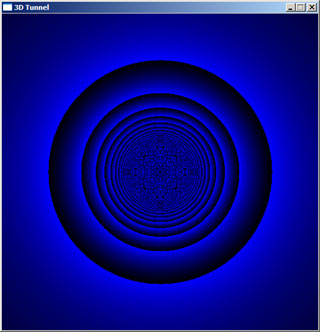### Calculating the depth

The y-coordinate of the texture is based on the depth to the pixel on the screen. This is easier code-wise to calculate, but isn't as immediately obvious maths-wise.

Essentially, you take a big number (such as the texture width) and divide it by the distance squared in the plane of screen to each pixel from the centre. That no doubt sounds a bit confusing - what I mean, really, is that the depth is:

depth = big_number / (x2+y2)

We then plug that depth value straight in as the y-coordinate of the texture. For a 512x512 pixel display, a "big_number" of 8388608 (223) appears to work as a "sensible" value. The sample pseudo-code shows how easy this stage is:

```for y is 0 to screen_height - 1
for x is 0 to screen_width - 1

relative_x = x - screen_width / 2
relative_y = screen_height / 2 - y

depth = 8388608 / (relative_x^2 + relative_y^2)

depth_lut(x,y) = depth

next x
next y```

Test this stage in the same way as you did for the angle table - make sure that you keep the value in the range for your pixel-plotter though! (The values should be positive, so in a C-type language you can just use the % operator to keep it valid).You should end up with something like the above image. You will no doubt notice the horrible moiré patterns in the distance - we'll fix those later. As before, the major problem here is going to be casting to and from integers and doubles/floats. Keeping everything in floating-point maths until the final cast into our lookup tables is a good plan - after all, it's just a one-off batch of calculations at the start of the program!